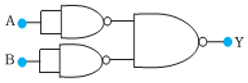(a) With proper diagram, explain the movement of charge carries through

different parts of the transistor and hence show that ${\mathbf{I}}_{\mathbf{E}}\mathbf{=}{\mathbf{I}}_{\mathbf{B}}\mathbf{+}{\mathbf{I}}_{\mathbf{c}}\mathbf{.}$

(b) Identify the logic operation carried out by the circuit shown below and

write its truth table.